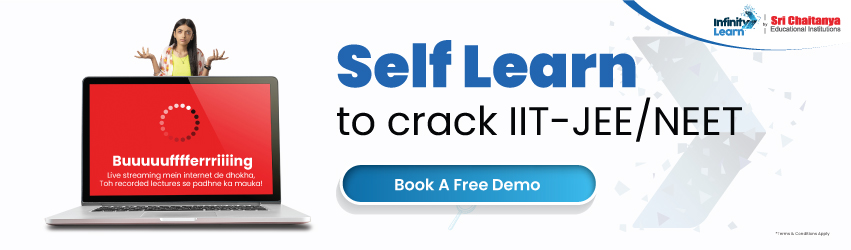Previous Year Papers Questions – JEE Main Mathematics Quadratic Equations And Expressions

# Previous Year Papers Questions – JEE Main Mathematics Quadratic Equations And Expressionsinfinitylearn surge self learn
infinitylearn starter package

## JEE Main Previous Year Papers Questions With Solutions

Get Previous Year Questions for JEE Main on Infinity Learn for free.

Need FREE NCERT/CBSE/IIT-JEE/NEET Study Material?

### Mathematics Quadratic Equations And ExpressionsAns.Ans.Ans.Ans.(1) p + q = -p and pq = q => q(p-1)=0 => q=0 or p=1.
If q = 0, then p = 0. i.e., p – q\p = 1 and q = -2.Ans.Ans.Ans.Ans.Ans.Ans.Ans.Ans.Ans.Ans.Ans.Ans.Ans.Ans.Ans.Ans.Ans.Ans.Ans.Ans.Join Infinity Learn Regular Class Programme!